#CIS 307: Deadlocks

## Introduction

Deadlock occurs when we have a set of processes, each holding some resources, each requesting some resources, and none of them is able to obtain what it needs, i.e. to make progress. We will usually reason in terms of resources R1, R2, .., Rm and processes P1, P2, .., Pn.

Resources can be preemptable or non-preemptable. A resource is preemptable if it can be taken away from the process that is holding it [we can think that the original holder waits, frozen, until the resource is returned to it]. Memory is an example of a preemptable resource. Of course, one may choose to deal with intrinsically preemptable resources as if they were non-preemptable. In our discussion we only consider non-preemptable resources.
Resources can be reusable or consumable. They are reusable if they can be used again after a process is done using them. Memory, printers, tape drives are examples of reusable resources. Consumable resources are resources that can be used only once. For example a message or an event. If two processes are waiting for a message and one receives it, then the other process remains waiting. To reason about deadlocks when dealing with consumable resources is extremely difficult. Thus we will restrict our discussion to reusable resources.
Resources are usually with a multiplicity, i.e. an indication of how many copies of the resource exist. So we may have 3 tape drives, 2 printers, etc. We normally assume that resources have a multiplicity different than 1. If it were always 1 the study of deadlocks could be simplified.

## Resource Allocation Graphs

Resource Allocation Graphs (RAGs) are directed labeled graphs used to represent, from the point of view of deadlocks, the current state of a system.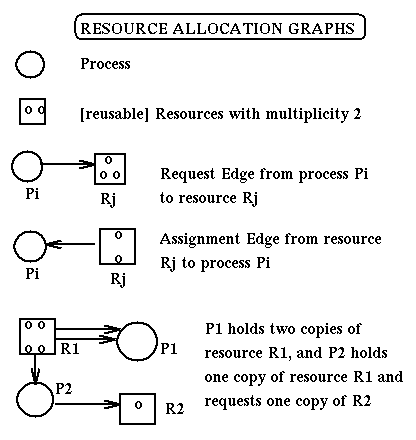State transitions can be represented as transitions between the corresponding resource allocation graphs. Here are the rules for state transitions:

• REQUEST: if process Pi has no outstanding request, it can request simultaneously any number (up to multiplicity) of resources R1, R2, ..Rm. The request is represented by adding appropriate requests edges to the RAG of the current state.
• ACQUISITION: if process Pi has outstanding requests and they can all be simultaneously satisfied, then the request edges of these requests are replaced by assignment edges in the RAG of the current state
• RELEASE: if process Pi has no outstanding request then it can release any of the resources it is holding, and remove the corrisponding assignment edges from the RAG of the current state.

1. If a the RAG of a state of a system is fully reducible (i.e. it can be reduced to a graph without any edges using ACQUISITION and RELEASE operations) then that state is not a deadlock state.
2. If a state is not a deadlock state then its RAG is fully reducible [this holds only if we are dealing with reusable resources; it is false if we have consumable resources]
3. A cycle in the RAG of a state is a necessary condition for that being a deadlock state
4. A cycle in the RAG of a state is a sufficient condition for that being a deadlock state only in the case of reusable resources with multiplicity one.

Here is an example of reduction of a RAG: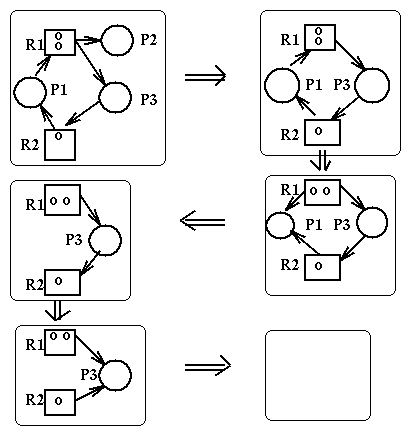## State Graphs

Where a resource allocation graph describes the current state of a system, a State Graph describes from the point of view of deadlocks all the states of a system.

Here is a system

```    P1:REPEAT                   P2:REPEAT
........                    ........
1    request(Disk);         1    request(Tape);
........                    ........
2    request(Tape);         2    request(Disk);
........                    ........
3    release(Tape);         3    release(Disk);
........                    ........
4    release(Disk)          4    release(Tape);
........                    ........
FOREVER                     FOREVER
```
Here is the corresponding State Graph [the state of a program when starting to execute a statement is the line number of that statement; the state of a system is the ordered pair of the sates of the programs]: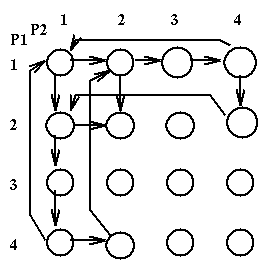Here we see that state [2,2] is a deadlock state. If we had recognised also a state1.5 between statements 1 and 2 we would have created a more complex state graph where some states would be semi-deadlock states, i.e. states where the system is not yet deadlocked but it will have no choice but to become deadlocked. In general we can have the following kinds of states:

• Secure: if no matter the operation we do we will not get deadlocked
• Safe: if there is a way to complete all processes without getting into a deadlock state
• Unsafe: if we will not be safe. It is further distinguished into semi-deadlock (we are not deadlocked, but we certainly will), deadlock (there are processes that are deadlocked), and total deadlock (all processes are deadlocked).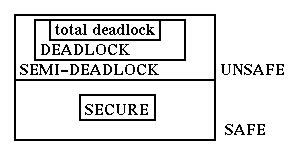Deadlock Prevention is to use resources in such a way that we cannot get into deadlocks. In real life we may decide that left turns are too dangerous, so we only do right turns. It takes longer to get there but it works. In terms of deadlocks, we may constrain our use of resources so that we do not have to worry about deadlocks. Here we explore this idea with two eaxmples.

### Linear Ordering of Resources

Assume that all resources are totally ordered from 1 to r. We may impose the following constraint:

• LO: A process cannot request a resource Rk if it is holding a resource Rh with k < h

It is easy to see that with this rule we will not get into deadlocks. [Proof by contradiction.]
Here is an example of how we apply this rule. We are given a process that uses resources ordered as A, B, C, D, E in the following manner: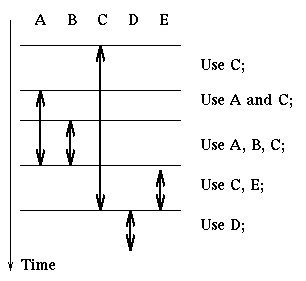Then the process can do the following:

```    acquire(A); acquire(B); acquire(C);
use C
use A and C
use A, B, C
release(A); release(B); acquire(E);
use C and E
release(C); release(E); acquire(D);
use D
release(D);
```
A strategy such as this can be used when we have a few resources. It is easy to apply and does not reduce the degree of concurrency too much.

### Hierarchical Ordering of Resources

Another strategy we may use in the case that resources are hierarchically structured is to lock them in hierarchical order. We assume that the resources are organized in a tree (or a forest) representing containment. We can lock any node or group of nodes in the tree. The resources we are interested in are nodes in the tree, usually leaves. Then the following rule will guarantee avoidance of deadlocks.

• HO: The nodes currently locked by a process must lay on all paths from the root to the desired resources.

Here is an example of use of this rule, locking a single resource at a time.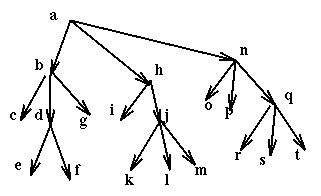Then if a process wants to use the resources e, f, i, k it uses in sequence the commands:

## Deadlock Avoidance and the Banker's Algorithm

Deadlock Avoidance, assuming that we are in a safe state and we are requested certain resources, simulates the allocation of those resources and determines if the resultant state is safe. If it is safe the request is satisfied, otherwise it is delaied until it becomes safe.

The Banker's Algorithm is used to determine if a request can be satisfied. It uses the following variables:

• AVAILABLE : array [1 .. m] of integer; -- it specifies for each resource how many copies of it are available
• ALLOCATION: array [1..n, 1..m] of integer; -- ALLOCATION[i,j] specifies the number of copies of resource j that are allocated to process i.
• MAXIM: array [1..n, 1..m] of integer; -- MAXIM[i,j] specifies the maximum number of copies of resource j that process i will use.
• NEED; array [1..n, 1..m] of integer; -- NEED[i,j] specifies the number of copies of resource j that process i still requires. It is equal to MAXIM[i,j]-ALLOCATION[i,j]
and the following notation
• A < B, where A and B are m-ary vectors, is true iff for all i, A[i] < B[i]
• If A is a rectangular matrix, Ai is its ith row.
```    procedure BANKER(REQUEST_I: array[1..m] of integer;
i : 1..n) is
{
if REQUEST_I > NEEDi then
ERROR;    -- The user is asking more than the agreed maximum
repeat
while (REQUEST_i > AVAILABLE)
yield; -- Resources are not available at this time
ALLOCATION_i = ALLOCATION_i + REQUEST_i;
AVAILABLE = AVAILABLE - REQUEST_I;
if SAFE_STATE then
RETURN;  -- The request is approved
ALLOCATION_i = ALLOCATION_I - REQUEST_i;
AVAILABLE = AVAILABLE + REQUEST_i;
YIELD;  -- The request cannot safely be satisfied at this time
forever;
}

BOOLEAN function SAFESTATE is -- Determines if current state is safe
{ NOCHANGE : boolean;
WORK : array[1..m] of INTEGER = AVAILABLE;
FINISH : array[1..n] of boolean = [false, ..,false];
I : integer;

repeat
NOCHANGE = TRUE;
for I = 1 to N do
if ((not FINISH[i]) and
NEEDi <= WORK) then {
WORK = WORK + ALLOCATION_i;
FINISH[i] = true;
NOCHANGE = false;
}
until NOCHANGE;
return (FINISH == (true, .., true));
}
```
The time complexity of the Banker's algorithm as a function of the number n of processes and m of resources is o(n*n*m).

Here is an example of use of the Banker's algorithm in the case of a single resource with multiplicity 12 and three processes, P1, P2, P3 which have a maximum need of, respectively, 10, 4, and 9. Currently these processes have respectively 5, 2, and 2 copies of the resource.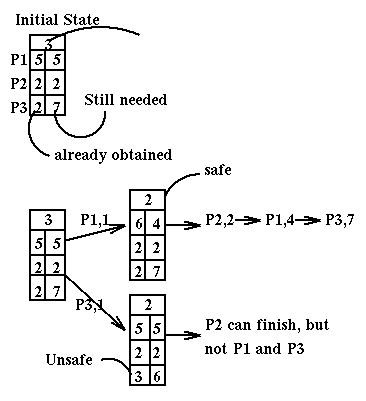Note that we can use the SafeState routine to determine if we are in a deadlock state. More precisely, it recognizes unsafe states, i.e. deadlock and almost-deadlock states. But this is what we are usually interested in instead of just deadlock states.

In practice deadlocks are dealt with in a variety of ways. Prevention (linear ordering) for internal resources like PCBs and buffers; prevention (pre-emption by swapping out) for central memory; avoidance (banker's algorithm) for job resources like tape drives; pre-allocation with given maxima for swapping space; or just the Ostrich Solution (don't worry about the possibility of deadlocks) if they are sufficiently rare.

ingargiola.cis.temple.edu